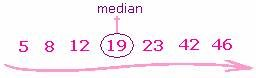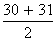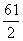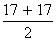## Definition Of Median

Median is the middle data value of an ordered data set.

If there are two middle values, then the median is the mean of the two numbers.
There will be two middle values when the number of values in the data set is even.

### Examples of Median

12, 23, 8, 46, 5, 42, 19
Mean =The median in the above data set is 19.

The median for the data set 2, 4, 7, 9, 3 is 4. 2, 3, 4, 7, and 9 is the ascending order of the data set 2, 4, 7, 9, 3. The middle number in the ordered data set is 4.

Let us find the median of a data set with even number of items in it, e.g. 33, 30, 42, 22, 18, and 31.
Arranging the above data set in ascending order, we find 18, 22, 30, 31, 33, and 42.
The middle numbers from the above data set are 30 and 31. As there are two middle numbers we have to find the mean of those numbers.== 30.5. So, 30.5 is the median (middle value) of the data set 33, 30, 42, 22, 18, and 31.

### Solved Example on Median

#### Ques: The given data shows the number of burgers sold at a bakery in the last 14 weeks. 17, 13, 18, 17, 13, 16, 18, 19, 17, 13, 16, 18, 20, 19 Find the median number of burgers sold.

##### Choices:

A. 18.5
B. 17
C. 18
D. 17.5
Step 5: Median == 17